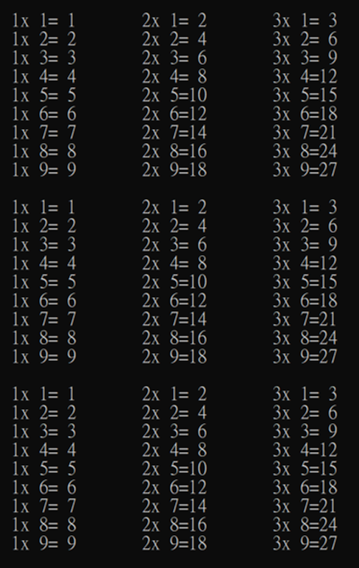WFU

## 2021年7月10日 星期六

### C語言練習01：九九乘法表#include<stdio.h>
int main(){
int k,i,j;
for(k = 1; k < 4; k++){
for(j = 1; j < 10; j++){
for(i = 3 * k - 2; i < 3 * k + 1; i++){
}
printf("\n");
}
printf("\n");
}
return 0;
}

1x 1= 1    =>    2x 1= 2    =>    3x 1= 3    =>    1x 2= 2    =>    2x 2= 4    這樣子下去...

4x 1= 4    =>    5x 1= 5    =>    5x 1= 5    =>    4x 2= 8    =>    5x 2=10......

7x 1= 7    =>    8x 1= 8    =>    9x 1= 9    =>    7x 2=14    =>    8x 2=16.....

for (k = 0; k < 4; k++){}就是在說k只能小於4（k只能是整數），也就是k最多為3，所以當k被加到3為止就會停了，而k一開始是0，所以從k = 0開始，每次加1，總共要加3次，所以從k是0到k是3的時間，就會讓for loop裡面的程式執行3次，這就是最外圈for loop的功用。